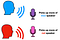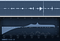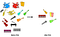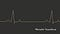# Independent Component Analysis (ICA)

## Finding hidden factors in data

This is the final post in a two-part series on Principal Component Analysis (PCA) and Independent Component Analysis (ICA). Although the techniques are similar, they are in fact different approaches and perform different tasks. In this post, I will provide a high level introduction to ICA, compare it to PCA, and give an example of using ICA to remove blink artifacts from EEG data.

More resources on this topic: Youtube video and example codeSimplest version of the “Cocktail Party Problem”. Image by author.

# ICA

The standard problem used to describe ICA is the “Cocktail Party Problem”. In its simplest form, imagine two people having a conversation at a cocktail party…

# The Fast Fourier Transform (FFT)

## With a teaspoon of intuition

This is the second part of a 3-part series on Fourier and Wavelet Transforms. In this article I will describe the Fast-Fourier Transform (FFT) and attempt to give some intuition as to what makes it so fast. The key impact of FFT is it provides an efficient way to compute the Fourier Transform of real-world data. An example applying FFT to the audio signal of a guitar is presented.

More resources on this topic: Youtube video and example codeThe Fast Fourier Transform fosters key techniques in audio production. Here, audio is edited based on its frequency spectrum.

# Discrete Fourier Transform (DFT)

In my previous post the Fourier Transform (FT) and some applications were introduced. In signal processing, this formal FT definition is…

# Principal Component Analysis (PCA)

## Intuition, math, and stonks

This is the first post in a two-part series on Principal Component Analysis (PCA) and Independent Component Analysis (ICA). Although they have similarities (such as their names), they each achieve different tasks. In this post, I will give describe what PCA is, how it works, and as an example use it to define an S&P 500 index fund. Example code and other related resources can be found in the last section of this post.

More resources on this topic: Youtube video and example codeVisual analogy of PCA. Image by author.

# PCA

Imagine a big rock band with about 20 members. It features guitarists, background singers, pianists, keyboardists…

# The Wavelet Transform

## An Introduction and Example

This is the final post in a 3-part series on Fourier and Wavelet Transforms. In previous posts both the Fourier Transform (FT) and its practical implementation the Fast-Fourier Transform (FFT) are discussed. In this post a similar idea is introduced, the Wavelet Transform. Once you have a solid understanding of how the FT works, wrapping your head around the Wavelet Transform is straightforward. I finish this post with a concrete example to show just one of many possible applications.

More resources on this topic: Youtube video and example codeImage by author

# Wavelet Transform

A major disadvantage of the Fourier Transform is it captures global

# Time Series, Signals, & the Fourier Transform

## An introduction and primer for future posts

In my next three posts I will introduce and discuss the Fourier and Wavelet Transforms, which extract information on how a quantity oscillates over time. These methods have an almost endless number of applications, a few of which I describe here. Youtube video and example code links can be found in the “Complementing Resources” section below.

# Time Series

Everyone is undoubtedly familiar with time series, even if you have not heard the term. A time series is simply a set of values ordered by time. For instance, stock index prices, which are usually depicted as price vs time plots on your favorite…## Shawhin Talebi

Data Scientist | Researcher | PhD Candidate